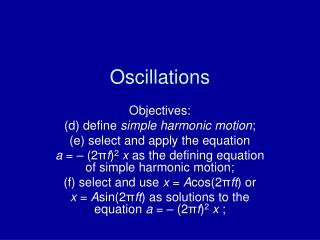DownloadDownload PresentationOscillations

# Oscillations

Télécharger la présentation## Oscillations

- - - - - - - - - - - - - - - - - - - - - - - - - - - E N D - - - - - - - - - - - - - - - - - - - - - - - - - - -
##### Presentation Transcript

1. Oscillations Objectives: (d) define simple harmonic motion; (e) select and apply the equation a = – (2πf)2x as the defining equation of simple harmonic motion; (f) select and use x = Acos(2πft) or x = Asin(2πft) as solutions to the equation a = – (2πf)2x ;

2. Outcomes All Must Be able to define simple harmonic motion. Most Should Be able to select and apply the equation a = – (2πf)2x as the defining equation of simple harmonic motion; Be able to select and use x = Acos(2πft) or x = Asin(2πft) as solutions to the equation a = – (2πf)2x. Be able to explain why the period of an object with simple harmonic motion is independent of its amplitude;

3. Oscillations • What is an oscillation? • An object oscillates when it moves repeatedly to and fro about a fixed point

4. Representing oscillations • We have demonstrated how an oscillation can be described in similar terms to circular motion

5. The Auxiliary Circle

6. Phase • Two different masses released at the same time • Would be completely in phase with each other: • 0 degrees out of phase, 0 radians.

7. Phase • Two different masses released at different times • Would be out of phase with each • other: ¼ of a cycle, 90 degrees out of phase, • or /2 radians.

8. Phase • Two different masses released at different times • Would be completely out of phase with each • other: ½ a cycle, 180 degrees out of phase, • or  radians.